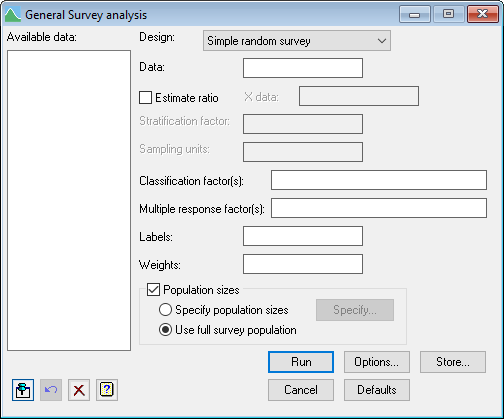1. Home
2. General Survey Analysis

# General Survey Analysis

Select menu: Stats | Survey Analysis | General Survey Analysis

Use this to calculate estimates from surveys, together with the asymptotic standard errors, for allowing the design of the survey.

Stats | Survey Analysis | General Survey Analysis.
2. Fill in the fields as required then click Run.

You can set additional Options before running the analysis and store the results by clicking Store.Information about the numbers of sampling units in the survey population is required and can be supplied in one of three ways.

1. The weights can be supplied in a variate. This will generally be the inverse of the probability of selection (pi expansion weights). However, this cannot convey the full design information for multi-stage surveys.
2. The population sizes can be specified by supplying the number of primary sampling units per stratum and for a two-stage design, the number of secondary units in each primary sampling unit. These options can be set by selecting the Population sizes and Specify population sizes options then clicking the Specify button.
3. Using the full survey population with unsampled (or non-responding) units indicated by missing values for the response variables. To use this method select the Population sizes and Use full survey population options.

## Available data

This lists data structures appropriate to the current input field. The contents will change as you move from one field to the next. You can double-click a name to copy it to the current input field or type it in.

## Design

Specifies the type of survey to tabulate. You can choose from a Simple random survey, a Stratified random survey, One stage cluster survey or Two-way survey.

## Data

This is the variate containing the survey data to be analysed. It should contain a value for each unit in the population, with unsampled or non-responding units being indicated by missing values.

## Estimate ratio

When selected, you can calculate estimates of the ratio Y/X by supplying a variate containing data for the denominator (X).

## Stratification factor

For a stratified survey, this is the factor indicating the stratum to which each unit belongs.

## Sampling units

A factor indicating the primary sampling units for a two stage survey or one stage cluster survey.

## Classification factor(s)

One or more factors separated by commas to define subsets of the data for which estimates are required. By default, estimates of totals, means or ratios are calculated for the whole population.

## Multiple response factor(s)

One or more pointers to a set of factors defining a set of multiple responses. If more than one pointer is used, they should be separated by commas. Multiple responses occur in surveys as the result of open-ended questions like “Which cities have you visited?“. You can form a pointer to factors for a multiple-response table using the form multiple-response factors menu. In Genstat, these can be formed using the FMFACTORS procedure and are represented by a pointer containing a factor for each possible response code. The factors have levels 0 and 1, and corresponding labels ‘absent’ and ‘present’. If the original response codes were textual, the various strings are used as labels of the pointer; while if they were numerical, the numbers are used as the pointer suffixes.

## Labels

This is an optional variate, factor or text which is used to label influential points. If it is left blank points are labelled by the numbers 1…n.

## Weights

An optional variate containing survey weights.

## Population sizes

When selected, information can be supplied about the numbers of sampling units. Select the Specify population sizes setting and click the Specify button to supply the number of primary sampling units per stratum, or, the number of secondary units per primary sampling unit (for a two-stage design). Alternatively, select the Use full survey population setting to use the full survey population where unsampled (or non-responding) units are indicated by missing values.

## Action IconsPin Controls whether to keep the dialog open when you click Run. When the pin is downthe dialog will remain open, otherwise when the pin is upthe dialog will close.Restore Restore names into edit fields and default settings.Clear Clear all fields and list boxes.Help Open the Help topic for this dialog.